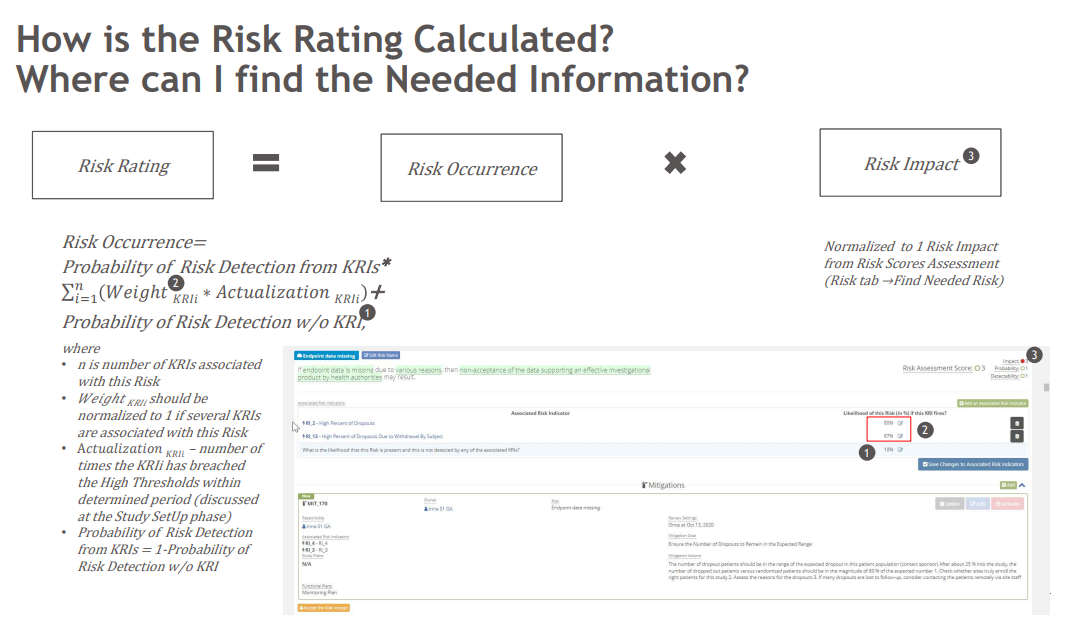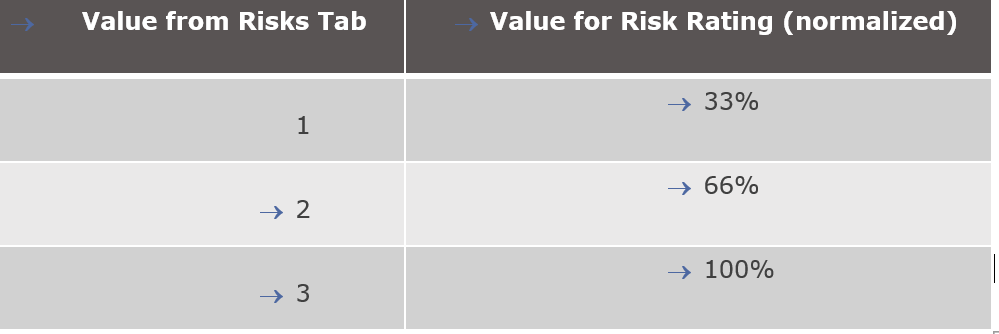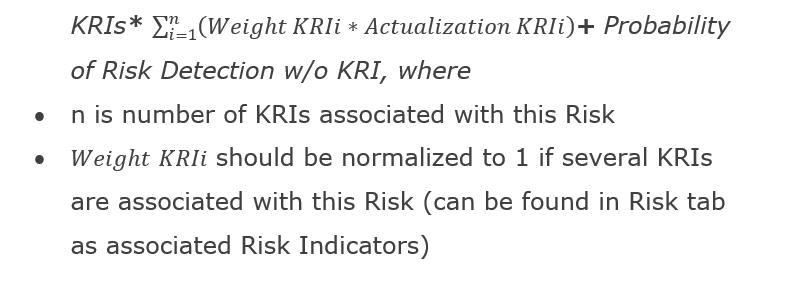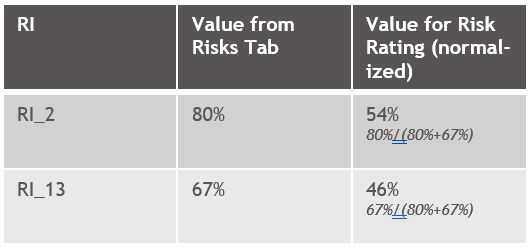# Risk Rating_The Algorithm of Calculation for the Risk RatingDetails of normalization

1.   Risk rating is a result of Risk Occurrence multiplication by Risk Impact

2.   Risk Impact should be taken from Risk tab and normalized to 13. Risk Occurrence= Probability of Risk Detection fromExample of normalization:Probability of  Risk Detection from KRIs = 1-Probability of Risk Detection w/o KRI